## Example Questions

### Example Question #1 : Quadrilaterals

ABCD is a parallelogram. BD = 5. The angles of triangle ABD are all equal. What is the perimeter of the parallelogram?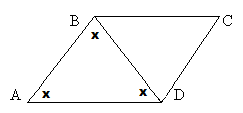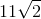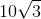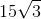Explanation:

If all of the angles in triangle ABD are equal and line BD divides the parallelogram, then all angles in triangle BDC must be equal as well.

We now have two equilateral triangles, so all sides of the triangles will be equal.

All sides therefore equal 5.

5+5+5+5 = 20

### Example Question #5 : Quadrilaterals

Find the area of a parallelogram with length 6 and height 5.Explanation:

To solve, simply use the formula for the area of a parallelogram. Thus,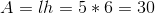Don't let a parallelogram fool you. It is just like a rectangle but on a slant. As long as you are given the height, and not the slant side length, you can use the same formula.

### Example Question #1 : Parallelograms

Find the perimeter of a parallelogram with one side equal to 6 and the other to 5.Explanation:

To solve, simply use the formula for the perimeter of a parallelogram. Thus,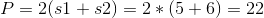If you don't remember this formula, you can also just sum up all the sides of the parallelogram.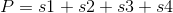Where s1 and s3 are the long sides and s2 and s4 are the short sides. Since the two pairs of sides are equal, you can sub in the following.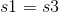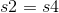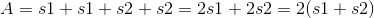### All SAT Math Resources